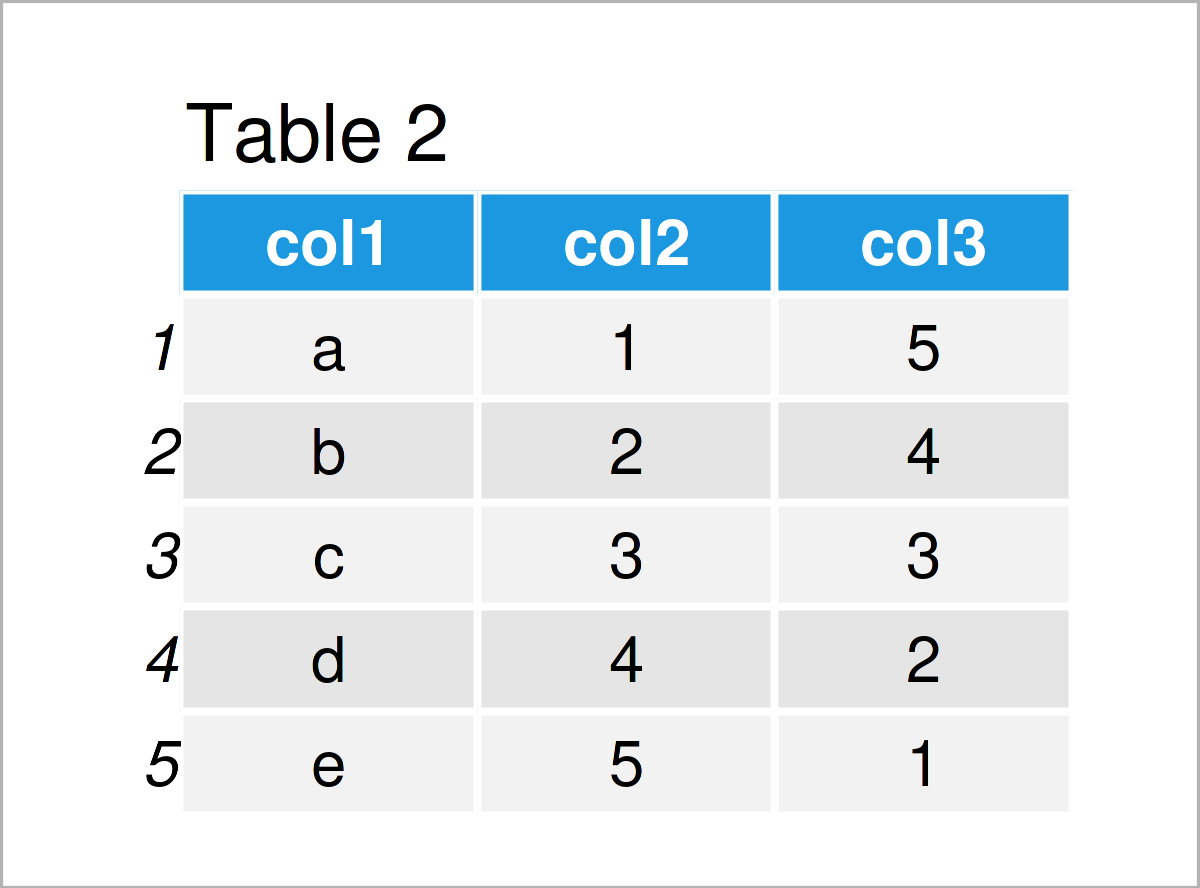# Add New Column to Front of Data Frame in R (2 Examples)

On this page you’ll learn how to add a new variable to the first index position of a data frame in the R programming language.

Sound good? Let’s get started…

## Creation of Example Data

Consider the following example data:

```data <- data.frame(col2 = 1:5,             # Create example data frame
col3 = 5:1)
data                                       # Print example data frame```As you can see based on Table 1, our example data is a data frame containing five rows and two variables called col2 and col3.

Furthermore, we have to create a vector object that we can append as a new column to the front of our data frame later on:

```new_col <- letters[1:5]                    # Create vector object
new_col                                    # Print vector object
#  "a" "b" "c" "d" "e"```

Looks good, let’s add this vector as a new column!

## Example 1: Add New Variable to First Index Position of Data Frame Using data.frame() Function

Example 1 illustrates how to use the data.frame function to append a vector object as a new variable at the beginning of a data frame.

For this, we can use the R syntax below:

```data_new1 <- data.frame(col1 = new_col,    # Append new column to front of data
data)
data_new1                                  # Print new data frame```As shown in Table 2, the previous syntax has managed to create a new data frame that contains our example vector as a new column at the front (not the end) of our data frame.

## Example 2: Add New Variable to First Index Position of Data Frame Using cbind() Function

Example 2 shows how to apply the cbind function to add a new column at the front of a data frame.

Consider the R code below:

```data_new2 <- cbind(col1 = new_col,         # Append new column to front of data
data)
data_new2                                  # Print new data frame```The output of the previous syntax is shown in Table 3. As you can see, we have constructed the same data frame as in Example 1. However, this time we have used the cbind function instead of the data.frame function.

## Video & Further Resources

If you need more explanations on the examples of the present post, you may want to watch the following video which I have published on my YouTube channel. I’m explaining the R syntax of this article in the video:

In addition, you might want to read some of the related tutorials on https://www.statisticsglobe.com/. Please find some related posts about similar topics such as loops, indices, and variables below:

In summary: In this post, I have demonstrated how to add a new column to the front (not the end) of a data frame in the R programming language. Kindly let me know in the comments below, in case you have any further questions.

Subscribe to the Statistics Globe Newsletter